# Equivalence of Bayesian priors and Ridge regression

Today I’m going to take you through the comparison of a Bayesian formalism for regression and compare it to Ridge regression which is a penalized version of OLS. The rationale I have for doing so is that many times in my career I’ve come across “frequentists” who claim that parameters can be controlled via a process called shrinkage, regularization, weight decay, or weight elimination depending on whether you’re using GLM’s, SVM’s or Neural networks. This statement is in principle correct while misguided. The regularization can be seen to arise as a consequence of a probabilistic formulation. I would go so far as to say that there is no such thing as frequentist statistics; there are only those who refuse to add prior information to their model! Before we get started I would like to warn you that this post is going to get a tad mathematical. If that scares you, you might consider skipping the majority of this post and go directly to the summary. Now, let’s go!

## A probabilistic formulation

Any regression problem can be expressed as an implementation of a probabilistic formulation. For instance what we typically have at our hand is a dependent variable y, a matrix X of covariates and a parameter vector β. The dependent variable consists of data we would like to learn something about or be able to explain. As such we wish to model it’s dynamics via the β through X. The joint probability distribution for these three ingredients is given simply as p(y, X,β). This is the most general form of representing a regression problem probabilistically. However, it’s not very useful, so in order to make it a bit more tangible let’s decompose this joint probability like this.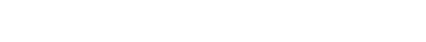In this view it is clear that we want to learn something about since that’s the unknowns. The other parts we have observed data on. So we would like to say something clever about p(β| y, X). How do we go about doing that? Well for starters we need to realize that p(y, X, β) can actually be written as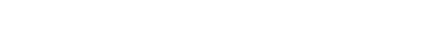which means that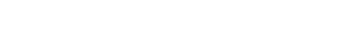and thereforwhich is just a derivation of Baye’s rule. Now we actually have something a bit more useful at our hands which is ready to be interpreted and implemented. What do I mean by implemented? Seems like an odd thing to say about probability distributions right? As weird as it may seem we actually haven’t given the probability distributions a concise mathematical representation. This is of course necessary for any kind of inference. So let’s get to it. The first term I would like to describe is the likelihood i.e. the p(y|β, X) which describes the likelihood of observing the data given the covariance matrix and a set of parameters β. For simplicity let’s say this probability distribution is gaussian thus taking the following form p(y|β, X) = N(y-βX; 0,σ). This corresponds to setting up a measurement model yt=βxt + ????where ???? ~ N(0,σ).

The second term in the nominator on the right hand side is our prior p(β) which we will also consider gaussian. Thus, we will set p(β)=N(0,αI) indicating that the parameters are independant from each other and most likely centered around with 0 a known standard deviation of α. The last term is the denominator p(y) which in this setting functions as the evidence. This is also the normalizing constant that makes sure that we can interpret the right hand side probabilistically.

That’s it! We now have the pieces we need to push the inference button. This is often for more complicated models done by utilizing Markov Chain Monte Carlo methods to sample the distributions. If we are not interested in the distribution but only the average estimates for the parameters we can just turn this into an optimization problem instead by realizing thatsince p(y) just functions as a normalizing constant and doesn’t change the location of the β that would yield the maximum probability. Thus we can set up the optimization problem asand maximize this function. Normally when we solve optimization problems it’s easier and nicer to turn it into a minimization problem instead of a maximization problem. This is easily done by minimizingas opposed to the equation before. For the sake of clarity let’s assume from now on that we only have one independent variable and only one parameter β. Since we know thatwe can easily unfold the logarithms to revealwhich can be more nicely written aswhere C=TC1-C2. As such putting a Gaussian prior on your β is equivalent to penalizing solutions that differ from 0 by a factor of 1/(2σ^2) i.e. 1 divided by two times the variance of the Gaussian. Thus, the smaller the variance, the higher our prior confidence is that the solution should be close to zero. The larger the variance the more uncertain we are about where the solution should end up.

## Ridge regression

The problem of regression can be formulated differently than we did previously, i.e., we don’t need to formulate it probabilistically. In essence what we could do is a state that we have a set of independent equations that we would like to solve like this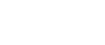where the variables and parameters have the same interpretation as before. This is basically Ordinary Least Squares (OLS) which suffers from overfitting and sensitivity to outliers and multicollinearity. So what Ridge regression does is to introduce a penalty term to this set of equations like this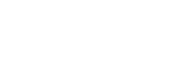where ???? is typically chosen to be γ????. This means that all values in the parameter vector β should be close to 0. Continuing along this track we can select a dumbed down version of this equation to show what’s going on for a simple application of one variable x and one parameter β. In this caseturns intowhich you may recognize from before. Not convinced? Well let’s look into the differences.Here it’s pretty obvious to see that they are equivalent. The constant C plays no role in the minimization of these expressions. Neither does the denominator 2σ^2. Thus if we set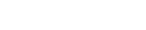the equivalence is clear and we see that what we are really minimizing iswhich concludes my point.

## Summary

So I’ve just shown that ridge regression and a Bayesian formulation with Gaussian priors on the parameters are in fact equivalent mathematically and numerically. One big question remains; Why the hell would anyone in their right mind use a probabilistic formulation for something as simple as penalized OLS? The answer you are looking for here is “freedom”. What if we would have selected a different likelihood? How about a different prior? All of this would have changed and we would have ended up with a different problem. The benefit of the probabilistic approach is that it is agnostic with respect to which distributions you choose. It’s a consistent inferential framework that just allows you the freedom to model things as you see fit. Ridge regression has already made all the model choices for you which is convenient but hardly universal.

My point is this; whatever model you decide to use and however you wish to model it is your prerogative. Embrace this freedom and don’t let old school convenient tools dictate your way towards solving a specific problem. Be creative, be free and most of all: Be honest to yourself.

Happy inferencing!

Dr. Michael Green
CEO, Co-Founder